# shapes

Submodule to generate basic geometric shapes.

## Arc

class vedo.shapes.Arc(center, point1, point2=None, normal=None, angle=None, invert=False, c='gray4', alpha=1, res=48)[source]

Bases: vedo.mesh.Mesh

Build a 2D circular arc between points point1 and point2. If normal is specified then center is ignored, and normal vector, a starting point1 (polar vector) and an angle defining the arc length need to be assigned.

Arc spans the shortest angular sector point1 and point2, if invert=True, then the opposite happens.

## Arrow

class vedo.shapes.Arrow(startPoint=(0, 0, 0), endPoint=(1, 0, 0), s=None, c='r4', alpha=1, res=12)[source]

Bases: vedo.mesh.Mesh

Build a 3D arrow from startPoint to endPoint of section size s, expressed as the fraction of the window size.

If c is a float less than 1, the arrow is rendered as a in a color scale from white to red.

Note

If s=None the arrow is scaled proportionally to its lengthtipPoint(returnIndex=False)[source]

Return the coordinates of the tip of the Arrow, or the point index.

## Arrow2D

class vedo.shapes.Arrow2D(startPoint=(0, 0, 0), endPoint=(1, 0, 0), shaftLength=0.8, shaftWidth=0.05, headLength=0.25, headWidth=0.2, fill=True, c='r4', alpha=1)[source]

Bases: vedo.mesh.Mesh

Build a 2D arrow from startPoint to endPoint.

Parameters
• shaftLength (float) – fractional shaft length

• shaftWidth (float) – fractional shaft width

• fill (bool) – if False only generate the outline

## Arrows

vedo.shapes.Arrows(startPoints, endPoints=None, s=None, thickness=1, c=None, alpha=1, res=12)[source]

Build arrows between two lists of points startPoints and endPoints. startPoints can be also passed in the form [[point1, point2], ...].

Color can be specified as a colormap which maps the size of the arrows.

Parameters
• s (float) – fix aspect-ratio of the arrow and scale its cross section

• c – color or color map name.

• alpha (float) – set transparency

• res (int) – set arrow resolution

## Arrows2D

Build 2D arrows between two lists of points startPoints and endPoints. startPoints can be also passed in the form [[point1, point2], ...].

Color can be specified as a colormap which maps the size of the arrows.

Parameters
• shaftLength (float) – fractional shaft length

• shaftWidth (float) – fractional shaft width

• fill (bool) – if False only generate the outline

• c – color

• alpha (float) – set transparency

Example
from vedo import Grid, Arrows2D
g1 = Grid(sx=1, sy=1)
g2 = Grid(sx=1.2, sy=1.2).rotateZ(4)
arrs2d = Arrows2D(g1, g2, c='jet')
arrs2d.show(axes=1, bg='white')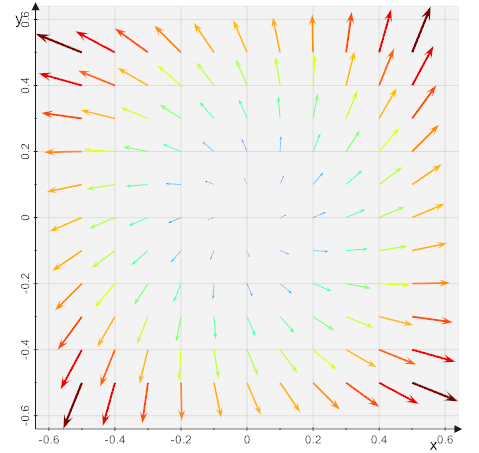## Bezier

vedo.shapes.Bezier(points, res=None)[source]

Generate the Bezier line that links the first to the last point.

Example
from vedo import *
import numpy as np
pts = np.random.randn(25,3)
for i,p in enumerate(pts):
p += [5*i, 15*sin(i/2), i*i*i/200]
show(Points(pts), Bezier(pts), axes=1)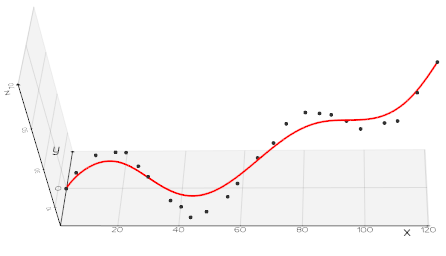## Box

class vedo.shapes.Box(pos=(0, 0, 0), length=1, width=2, height=3, size=(), c='g4', alpha=1)[source]

Bases: vedo.mesh.Mesh

Build a box of dimensions x=length, y=width and z=height. Alternatively dimensions can be defined by setting size keyword with a tuple. If size is a list of 6 numbers, this will be interpreted as the bounding box: [xmin,xmax, ymin,ymax, zmin,zmax]

## Brace

vedo.shapes.Brace(q1, q2, style='}', pad=0.2, thickness=1, font='Kanopus', comment='', s=1, c='k1', alpha=1)[source]

Create a brace (bracket) shape which spans from point q1 to point q2.

Parameters
• q1 (list) – point 1.

• q2 (list) – point 2.

• style (str, optional) – style of the bracket, eg. {}, [], (), <>. The default is ‘{‘.

• pad (float, optional) – padding space in percent. The default is 0.2.

• thickness (float, optional) – thickness factor for the bracket. The default is 1.

• font (str, optional) – font type. The default is ‘Kanopus’.

• comment (str, optional) – additional text to appear next to the bracket. The default is ‘’.

• s (float, optional) – scale factor for the comment

• scatter3.py (scatter3) –

## CSpline

class vedo.shapes.CSpline(points, closed=False, res=None)[source]

Return a Cardinal spline which runs exactly through all the input points.

Parameters
• closed (bool) – join last to first point to produce a closed curve

• res (int) – approximateresolution of the output line. Default is 20 times the number of input points.

See also: Spline and KSpline.

## Circle

class vedo.shapes.Circle(pos=(0, 0, 0), r=1, c='gray5', alpha=1, res=120)[source]

Build a Circle of radius r.

## Cone

class vedo.shapes.Cone(pos=(0, 0, 0), r=1, height=3, axis=(0, 0, 1), c='green3', alpha=1, res=48)[source]

Bases: vedo.mesh.Mesh

Build a cone of specified radius r and height, centered at pos.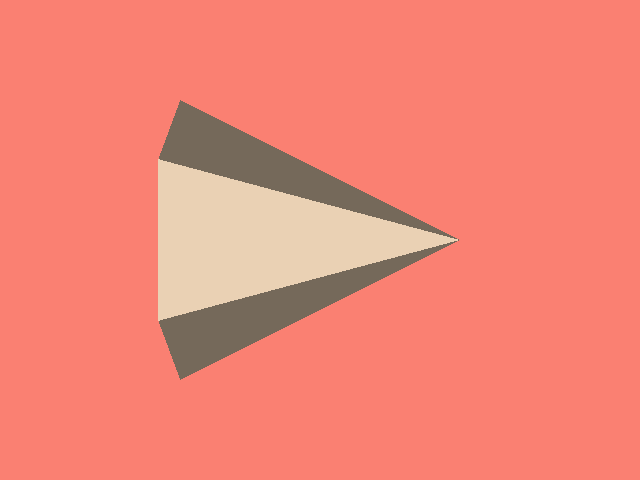## ConvexHull

vedo.shapes.ConvexHull(pts)[source]

Create the 2D/3D convex hull of a set of input points or input Mesh.

## CornerAnnotation

class vedo.shapes.CornerAnnotation(s=1, c=None, alpha=0.15, bg=None, font='')[source]

Bases: vtkmodules.vtkRenderingAnnotation.vtkCornerAnnotation, vedo.shapes.TextBase

Annotate the window corner with 2D text.

See Text2D description as the basic functionality is very similar.

The added value of this class is the possibility to manage with one single object the all corner annotations (instead of creating 4 Text2D instances).

See example: advanced/timer_callback2.py

clear()[source]
icon(pict)[source]
size(s, linear=False)[source]

The font size is calculated as the largest possible value such that the annotations for the given viewport do not overlap.

This font size can be scaled non-linearly with the viewport size, to maintain an acceptable readable size at larger viewport sizes, without being too big. f’ = linearScale * pow(f,nonlinearScale)

text(txt, pos=2)[source]

Set text at the assigned position

## Cross3D

vedo.shapes.Cross3D(pos=(0, 0, 0), s=1.0, thickness=0.3, c='b', alpha=1)[source]

Build a 3D cross shape, mainly useful as a 3D marker.

## Cube

vedo.shapes.Cube(pos=(0, 0, 0), side=1, c='g4', alpha=1)[source]

Build a cube of size side.

## Cylinder

class vedo.shapes.Cylinder(pos=(0, 0, 0), r=1, height=2, axis=(0, 0, 1), c='teal3', alpha=1, cap=True, res=24)[source]

Bases: vedo.mesh.Mesh

Build a cylinder of specified height and radius r, centered at pos.

If pos is a list of 2 Points, e.g. pos=[v1,v2], build a cylinder with base centered at v1 and top at v2.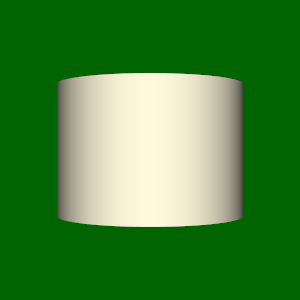## DashedLine

class vedo.shapes.DashedLine(p0, p1=None, spacing=0.1, closed=False, c='k5', alpha=1, lw=2)[source]

Build a dashed line segment between points p0 and p1. If p0 is a list of points returns the line connecting them. A 2D set of coords can also be passed as p0=[x..], p1=[y..].

Parameters
• closed (bool) – join last to first point

• spacing (float) – relative size of the dash.

• c (int, str, list) – color name, number, or list of [R,G,B] colors.

• alpha (float) – transparency in range [0,1].

• lw – line width.

## Disc

class vedo.shapes.Disc(pos=(0, 0, 0), r1=0.5, r2=1, c='gray4', alpha=1, res=12)[source]

Bases: vedo.mesh.Mesh

Build a 2D disc of inner radius r1 and outer radius r2.

Parameters

res (list) – resolution in R and Phi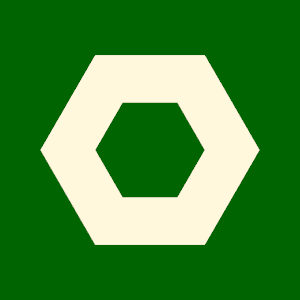## Earth

class vedo.shapes.Earth(style=1, r=1)[source]

Bases: vedo.mesh.Mesh

Build a textured mesh representing the Earth.

## Ellipsoid

class vedo.shapes.Ellipsoid(pos=(0, 0, 0), axis1=(1, 0, 0), axis2=(0, 2, 0), axis3=(0, 0, 3), c='cyan4', alpha=1, res=24)[source]

Bases: vedo.mesh.Mesh

Build a 3D ellipsoid centered at position pos.

Note

axis1 and axis2 are only used to define sizes and one azimuth angle.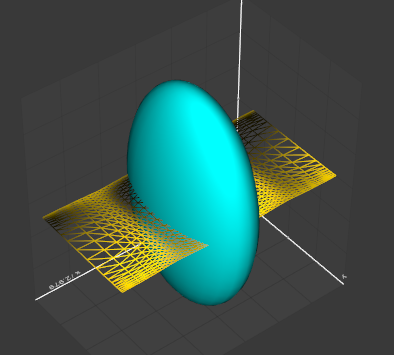asphericity()[source]

Return a measure of how different an ellipsoid is froma sphere. Values close to zero correspond to a spheric object.

asphericity_error()[source]

Calculate statistical error on the asphericity value.

Errors on the main axes are stored in Ellipsoid.va_error Ellipsoid.vb_error Ellipsoid.vc_error

## FlatArrow

vedo.shapes.FlatArrow(line1, line2, c='r4', alpha=1, tipSize=1, tipWidth=1)[source]

Build a 2D arrow in 3D space by joining two close lines.

## Glyph

class vedo.shapes.Glyph(mesh, glyphObj, orientationArray=None, scaleByScalar=False, scaleByVectorSize=False, scaleByVectorComponents=False, colorByScalar=False, colorByVectorSize=False, tol=0, c='k8', alpha=1)[source]

Bases: vedo.mesh.Mesh

At each vertex of a mesh, another mesh - a ‘glyph’ - is shown with various orientation options and coloring. The input mesh can also be a simple list of 2D or 3D coordinates. Color can be specified as a colormap which maps the size of the orientation vectors in orientationArray.

Parameters
• orientationArray (list, str, vtkAbstractArray) – list of vectors, vtkAbstractArray or the name of an already existing points array.

• scaleByScalar (bool) – glyph mesh is scaled by the active scalars.

• scaleByVectorSize (bool) – glyph mesh is scaled by the size of the vectors.

• scaleByVectorComponents (bool) – glyph mesh is scaled by the 3 vectors components.

• colorByScalar (bool) – glyph mesh is colored based on the scalar value.

• colorByVectorSize (bool) – glyph mesh is colored based on the vector size.

• tol (float) – set a minimum separation between two close glyphs (not compatible with orientationArray being a list).

## Grid

class vedo.shapes.Grid(pos=(0, 0, 0), normal=(0, 0, 1), sx=1, sy=1, sz=(0), c='k3', alpha=1, lw=1, resx=10, resy=10)[source]

Bases: vedo.mesh.Mesh

Return an even or uneven 2D grid at z=0.

Parameters
• sx (float,list) – if a float is provided it is interpreted as the total size along x, if a list of coords is provided they are interpreted as the vertices of the grid along x. In this case keyword resx is ignored (see example below).

• sy (float,list) – see above.

• lw (float) – line width.

• resx (int) – resolution along x, e.i. the number of axis subdivisions.

Example
from vedo import *
import numpy as np
xcoords = np.arange(0, 2, 0.2)
ycoords = np.arange(0, 1, 0.2)
sqrtx = sqrt(xcoords)
grid = Grid(sx=sqrtx, sy=ycoords)
grid.show(axes=8)


## Hyperboloid

class vedo.shapes.Hyperboloid(pos=(0, 0, 0), a2=1, value=0.5, height=1, c='pink4', alpha=1, res=100)[source]

Bases: vedo.mesh.Mesh

Build a hyperboloid of specified aperture a2 and height, centered at pos.

Full volumetric expression is:

$$F(x,y,z)=a_0x^2+a_1y^2+a_2z^2+a_3xy+a_4yz+a_5xz+ a_6x+a_7y+a_8z+a_9$$

## KSpline

class vedo.shapes.KSpline(points, continuity=0, tension=0, bias=0, closed=False, res=None)[source]

Return a Kochanek spline which runs exactly through all the input points.

Parameters
• continuity (float) – changes the sharpness in change between tangents

• tension (float) – changes the length of the tangent vector

• bias (float) – changes the direction of the tangent vector

• closed (bool) – join last to first point to produce a closed curve

• res (int) – approximate resolution of the output line. Default is 20 times the number of input points.

See also: Spline and CSpline.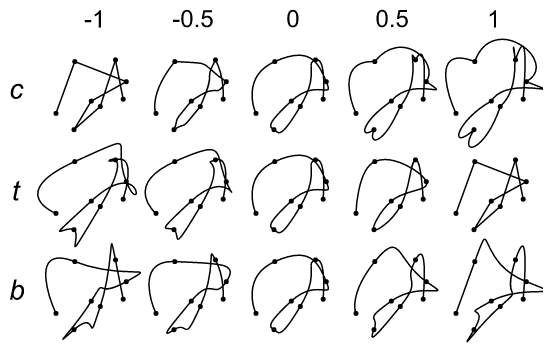## Latex

class vedo.shapes.Latex(formula, pos=(0, 0, 0), c='k', s=1, bg=None, alpha=1, res=30, usetex=False)[source]

Render Latex formulas.

Parameters
• formula (str) – latex text string

• pos (list) – position coordinates in space

• c – face color

• bg – background color box

• res (int) – dpi resolution

• usetex (bool) – use latex compiler of matplotlib

You can access the latex formula in Latex.formula’.

## Line

class vedo.shapes.Line(p0, p1=None, closed=False, c='k4', alpha=1, lw=1, res=2)[source]

Bases: vedo.mesh.Mesh

Build the line segment between points p0 and p1. If p0 is a list of points returns the line connecting them. A 2D set of coords can also be passed as p0=[x..], p1=[y..].

Parameters
• closed (bool) – join last to first point

• c (int, str, list) – color name, number, or list of [R,G,B] colors.

• alpha (float) – transparency in range [0,1].

• lw – line width.

• res (int) – resolution, number of points along the line (only relevant if only 2 points are specified)

eval(x)[source]

Calculate the position of an intermediate point as a fraction of the length of the line, being x=0 the first point and x=1 the last point. This corresponds to an imaginary point that travels along the line at constant speed.

Can be used in conjunction with linInterpolate() to map any range to the [0,1] range.

length()[source]

Calculate length of the line.

sweep(direction=(1, 0, 0), res=1)[source]

Sweep the Line along the specified vector direction.

Returns a Mesh surface. Line position is updated to allow for additional sweepings.

Example
from vedo import Line, show
aline = Line([(0,0,0),(1,3,0),(2,4,0)])
surf1 = aline.sweep((1,0.2,0), res=3)
surf2 = aline.sweep((0.2,0,1))
aline.color('r').lineWidth(4)
show(surf1, surf2, aline, axes=1)


## Lines

class vedo.shapes.Lines(startPoints, endPoints=None, c='k4', alpha=1, lw=1, dotted=False, scale=1, res=1)[source]

Build the line segments between two lists of points startPoints and endPoints. startPoints can be also passed in the form [[point1, point2], ...].

Parameters

scale (float) – apply a rescaling factor to the lengths.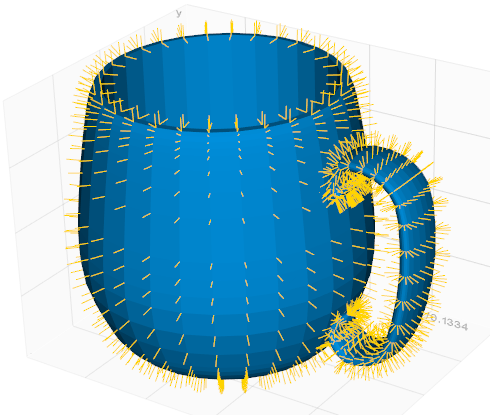## Marker

vedo.shapes.Marker(symbol, pos=(0, 0, 0), c='lb', alpha=1, s=0.1, filled=True)[source]

Generate a marker shape. Can be used in association with Glyph.

## NormalLines

vedo.shapes.NormalLines(mesh, ratio=1, atCells=True, scale=1)[source]

Build an Mesh made of the normals at cells shown as lines.

if atCells is False normals are shown at vertices.

## Paraboloid

class vedo.shapes.Paraboloid(pos=(0, 0, 0), r=1, height=1, c='cyan5', alpha=1, res=50)[source]

Bases: vedo.mesh.Mesh

Build a paraboloid of specified height and radius r, centered at pos.

Note

Full volumetric expression is:

$$F(x,y,z)=a_0x^2+a_1y^2+a_2z^2+a_3xy+a_4yz+a_5xz+ a_6x+a_7y+a_8z+a_9$$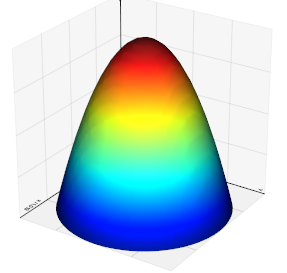## ParametricShape

class vedo.shapes.ParametricShape(name, res=51, n=25, seed=1)[source]

Bases: vedo.mesh.Mesh

A set of built-in shapes mainly for illustration purposes.

Name can be an integer or a string in this list:

[‘Boy’, ‘ConicSpiral’, ‘CrossCap’, ‘Dini’, ‘Enneper’, ‘Figure8Klein’, ‘Klein’, ‘Mobius’, ‘RandomHills’, ‘Roman’, ‘SuperEllipsoid’, ‘BohemianDome’, ‘Bour’, ‘CatalanMinimal’, ‘Henneberg’, ‘Kuen’, ‘PluckerConoid’, ‘Pseudosphere’].

Example
from vedo import *
for i in range(18):
ps = ParametricShape(i, c=i)
show([ps, ps.name], at=i, N=18)
interactive()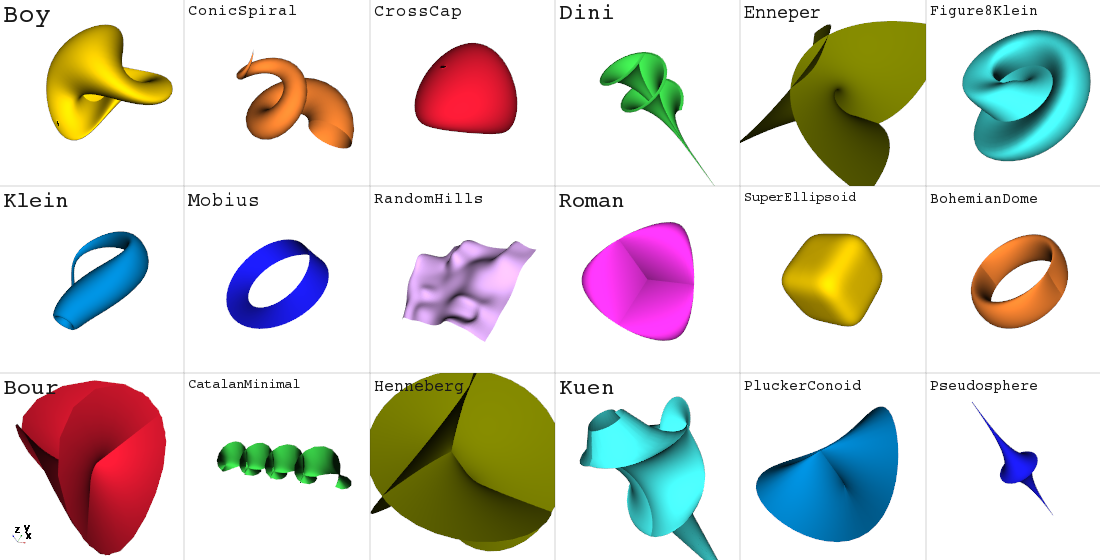## Plane

class vedo.shapes.Plane(pos=(0, 0, 0), normal=(0, 0, 1), sx=1, sy=None, c='gray6', alpha=1)[source]

Bases: vedo.mesh.Mesh

Draw a plane of size sx and sy oriented perpendicular to vector normal and so that it passes through point pos.## Polygon

class vedo.shapes.Polygon(pos=(0, 0, 0), nsides=6, r=1, c='coral', alpha=1)[source]

Bases: vedo.mesh.Mesh

Build a polygon in the xy plane of nsides of radius r.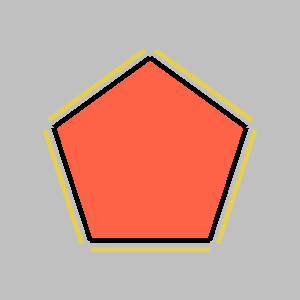## Pyramid

class vedo.shapes.Pyramid(pos=(0, 0, 0), s=1, height=1, axis=(0, 0, 1), c='green3', alpha=1)[source]

Build a pyramid of specified base size s and height, centered at pos.

## Rectangle

vedo.shapes.Rectangle(p1=(0, 0), p2=(2, 1), c='gray6', alpha=1)[source]

Build a rectangle in the xy plane identified by two corner points.

## Ribbon

class vedo.shapes.Ribbon(line1, line2=None, mode=0, closed=False, width=None, c='indigo3', alpha=1, res=(200, 5))[source]

Bases: vedo.mesh.Mesh

Connect two lines to generate the surface inbetween. Set the mode by which to create the ruled surface.

It also works with a single line in input. In this case the ribbon is formed by following the local plane of the line in space.

Parameters
• mode (int) – If mode=0, resample evenly the input lines (based on length) and generates triangle strips. If mode=1, use the existing points and walks around the polyline using existing points.

• closed (bool) – if True, join the last point with the first to form a closed surface

• res (list) – ribbon resolutions along the line and perpendicularly to it.

## RoundedLine

vedo.shapes.RoundedLine(pts, lw, c='gray4', alpha=1, res=10)[source]

Create a 2D line of specified thickness (in absolute units) passing through a list of input points. Borders of the line are rounded.

Parameters
• pts (list) – a list of points in 2D or 3D (z will be ignored).

• lw (float) – thickness of the line.

• res (int, optional) – resolution of the rounded regions. The default is 10.

Example

from vedo import *
pts = [(-4,-3),(1,1),(2,4),(4,1),(3,-1),(2,-5),(9,-3)]
ln = Line(pts, c='r', lw=2).z(0.01)
rl = RoundedLine(pts, 0.6)
show(Points(pts), ln, rl, axes=1)


## Sphere

class vedo.shapes.Sphere(pos=(0, 0, 0), r=1, c='r5', alpha=1, res=24, quads=False)[source]

Bases: vedo.mesh.Mesh

Build a sphere at position pos of radius r.

Parameters
• float (r) – sphere radius

• res (int) – resolution in phi, resolution in theta is 2*res

## Spheres

class vedo.shapes.Spheres(centers, r=1, c='r5', alpha=1, res=8)[source]

Bases: vedo.mesh.Mesh

Build a (possibly large) set of spheres at centers of radius r.

Either c or r can be a list of RGB colors or radii.

## Spline

class vedo.shapes.Spline(points, smooth=0, degree=2, closed=False, s=2, res=None, easing='')[source]

Find the B-Spline curve through a set of points. This curve does not necessarly pass exactly through all the input points. Needs to import scipy.

Return an Mesh object.

Parameters
• smooth (float) –

smoothing factor.

• 0 = interpolate points exactly [default].

• 1 = average point positions.

• degree (int) – degree of the spline (1<degree<5)

• easing (str) –

control sensity of points along the spline.

Available options are [InSine, OutSine, Sine, InQuad, OutQuad, InCubic, OutCubic, InQuart, OutQuart, InCirc, OutCirc]. Can be used to create animations (move objects at varying speed). See e.g.: https://easings.net

• res (int) – number of points on the spline

See also: CSpline and KSpline.

## Spring

class vedo.shapes.Spring(startPoint=(0, 0, 0), endPoint=(1, 0, 0), coils=20, r=0.1, r2=None, thickness=None, c='gray5', alpha=1)[source]

Bases: vedo.mesh.Mesh

Build a spring of specified nr of coils between startPoint and endPoint.

Parameters
• coils (int) – number of coils

• r (float) – radius at start point

• r2 (float) – radius at end point

• thickness (float) – thickness of the coil section

## Star

class vedo.shapes.Star(pos=(0, 0, 0), n=5, r1=0.7, r2=1.0, line=False, c='blue6', alpha=1)[source]

Bases: vedo.mesh.Mesh

Build a 2D star shape of n cusps of inner radius r1 and outer radius r2.

Parameters

line (bool) – only build the outer line (no internal surface meshing).

## Star3D

class vedo.shapes.Star3D(pos=(0, 0, 0), r=1.0, thickness=0.1, c='blue4', alpha=1)[source]

Bases: vedo.mesh.Mesh

Build a 3D star shape of 5 cusps, mainly useful as a 3D marker.

## Tensors

class vedo.shapes.Tensors(domain, source='ellipsoid', useEigenValues=True, isSymmetric=True, threeAxes=False, scale=1, maxScale=None, length=None, c=None, alpha=1)[source]

Bases: vedo.mesh.Mesh

Geometric representation of tensors defined on a domain or set of points. Tensors can be scaled and/or rotated according to the source at eache input point. Scaling and rotation is controlled by the eigenvalues/eigenvectors of the symmetrical part of the tensor as follows:

For each tensor, the eigenvalues (and associated eigenvectors) are sorted to determine the major, medium, and minor eigenvalues/eigenvectors. The eigenvalue decomposition only makes sense for symmetric tensors, hence the need to only consider the symmetric part of the tensor, which is 1/2*(T+T.transposed()).

Parameters
• source (str) – preset type of source shape [‘ellipsoid’, ‘cylinder’, ‘cube’ or any specified Mesh]

• useEigenValues (bool) – color source glyph using the eigenvalues or by scalars.

• threeAxes (bool) –

if False scale the source in the x-direction, the medium in the y-direction, and the minor in the z-direction. Then, the source is rotated so that the glyph’s local x-axis lies along the major eigenvector, y-axis along the medium eigenvector, and z-axis along the minor.

If True three sources are produced, each of them oriented along an eigenvector and scaled according to the corresponding eigenvector.

• isSymmetric (bool) – If True each source glyph is mirrored (2 or 6 glyphs will be produced). The x-axis of the source glyph will correspond to the eigenvector on output.

• length (float) – distance from the origin to the tip of the source glyph along the x-axis

• scale (float) – scaling factor of the source glyph.

• maxScale (float) – clamp scaling at this factor.

## TessellatedBox

vedo.shapes.TessellatedBox(pos=(0, 0, 0), n=10, spacing=(1, 1, 1), c='k5', alpha=0.5)[source]

Build a cubic Mesh made o n small quads in the 3 axis directions.

Parameters
• pos (list) – position of the left bottom corner

• n (int) – number of subdivisions

• spacing (list) – size of the side of the single quad in the 3 directions

## Text

vedo.shapes.Text(*args, **kwargs)[source]

## Text2D

class vedo.shapes.Text2D(txt='', pos='top-left', s=1, c=None, alpha=0.1, bg=None, font='', justify='', bold=False, italic=False)[source]

Bases: vtkmodules.vtkRenderingCore.vtkActor2D, vedo.shapes.TextBase

Returns a 2D text object. All properties of the text, and the text itself, can be changed after creation (which is expecially useful in loops).

Parameters
• pos

text is placed in one of the 8 positions:

bottom-left bottom-right top-left top-right bottom-middle middle-right middle-left top-middle

If a pair (x,y) is passed as input the 2D text is place at that position in the coordinate system of the 2D screen (with the origin sitting at the bottom left).

• s (float) – size of text.

• bg – background color

• alpha (float) – background opacity

• justify (str) – text justification

• font (str) –

predefined available fonts are

• Arial

• Bongas

• Calco

• Comae

• Courier

• Glasgo

• Kanopus

• LionelOfParis

• LogoType

• Normografo

• Quikhand

• SmartCouric

• Theemim

• Times

• VictorMono

• More fonts at: https://vedo.embl.es/fonts/

A path to a .otf or .ttf font-file can also be supplied as input.

pos(pos='top-left', justify='')[source]

Set position of the text to draw. Keyword pos can be a string or 2D coordinates in the range [0,1], being (0,0) the bottom left corner.

size(s)[source]
text(txt=None)[source]

Set/get the input text string

## Text3D

class vedo.shapes.Text3D(txt, pos=(0, 0, 0), s=1, font='', hspacing=1.15, vspacing=2.15, depth=0, italic=False, justify='bottom-left', c=None, alpha=1, literal=False)[source]

Bases: vedo.mesh.Mesh

Generate a 3D polygonal Mesh representing a text string.

Can render strings like 3.7 10^9 or H_2 O with subscripts and superscripts. Most Latex symbols are also supported (e.g. mu_lambda). Symbols ~ ^ _ are reserved modifiers:

use ~ to add a short space, 1/4 of the default empty space, use ^ and _ to start up/sub scripting, a space terminates their effect.

Monospaced fonts are: Calco, Glasgo, SmartCouric, VictorMono, Justino. More fonts at: https://vedo.embl.es/fonts/

Parameters
• pos (list) – position coordinates in 3D space

• s (float) – size of text.

• depth (float) – text thickness.

• italic (bool,float) – italic font type (can be a signed float too).

• justify (str) – text justification as centering of the bounding box (bottom-left, bottom-right, top-left, top-right, centered).

• font (str) – available 3D-polygonized fonts are Bongas, Calco, Comae, Kanopus, Glasgo, LionelOfParis, LogoType, Normografo, Quikhand, SmartCouric, Theemim, VictorMono, VTK, Capsmall, Cartoons123, PlanetBenson, Vega, Justino, Spears, Meson. Default is Normografo, which can be changed using settings.defaultFont

• hspacing (float) – horizontal spacing of the font.

• vspacing (float) – vertical spacing of the font for multiple lines text.

• literal (bool) – if set to True will ignore modifiers like _ or ^

## TextBase

class vedo.shapes.TextBase[source]

Bases: object

Do not instantiate this.

alpha(value)[source]
angle(a)[source]

Orientation angle in degrees

background(color='k9', alpha=1)[source]

Text background. Set to None to disable it.

bold(value=True)[source]
c(color)[source]
color(c)[source]
font(font=None)[source]
frame(color='k1', lw=2)[source]
italic(value=True)[source]
lineOffset(lo)[source]

Set/Get the vertical offset (measured in pixels).

lineSpacing(ls)[source]

Set the extra spacing between lines, expressed as a text height multiplication factor.

Text shadowing. Set to None to disable it.

## Torus

class vedo.shapes.Torus(pos=(0, 0, 0), r=1, thickness=0.2, c='yellow3', alpha=1, res=30)[source]

Bases: vedo.mesh.Mesh

Build a torus of specified outer radius r internal radius thickness, centered at pos.

## Tube

class vedo.shapes.Tube(points, r=1, cap=True, c=None, alpha=1, res=12)[source]

Bases: vedo.mesh.Mesh

Build a tube along the line defined by a set of points.

Parameters